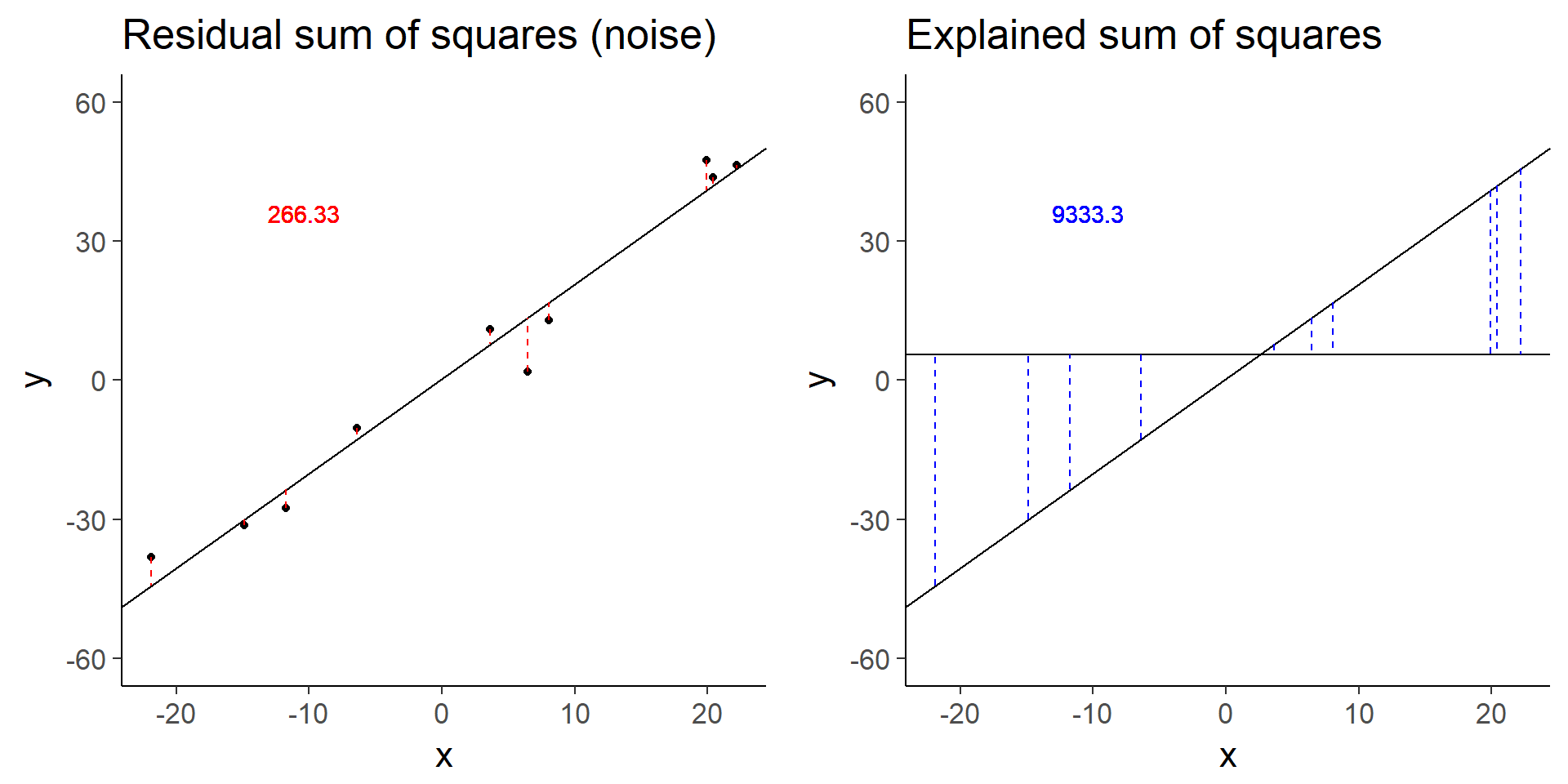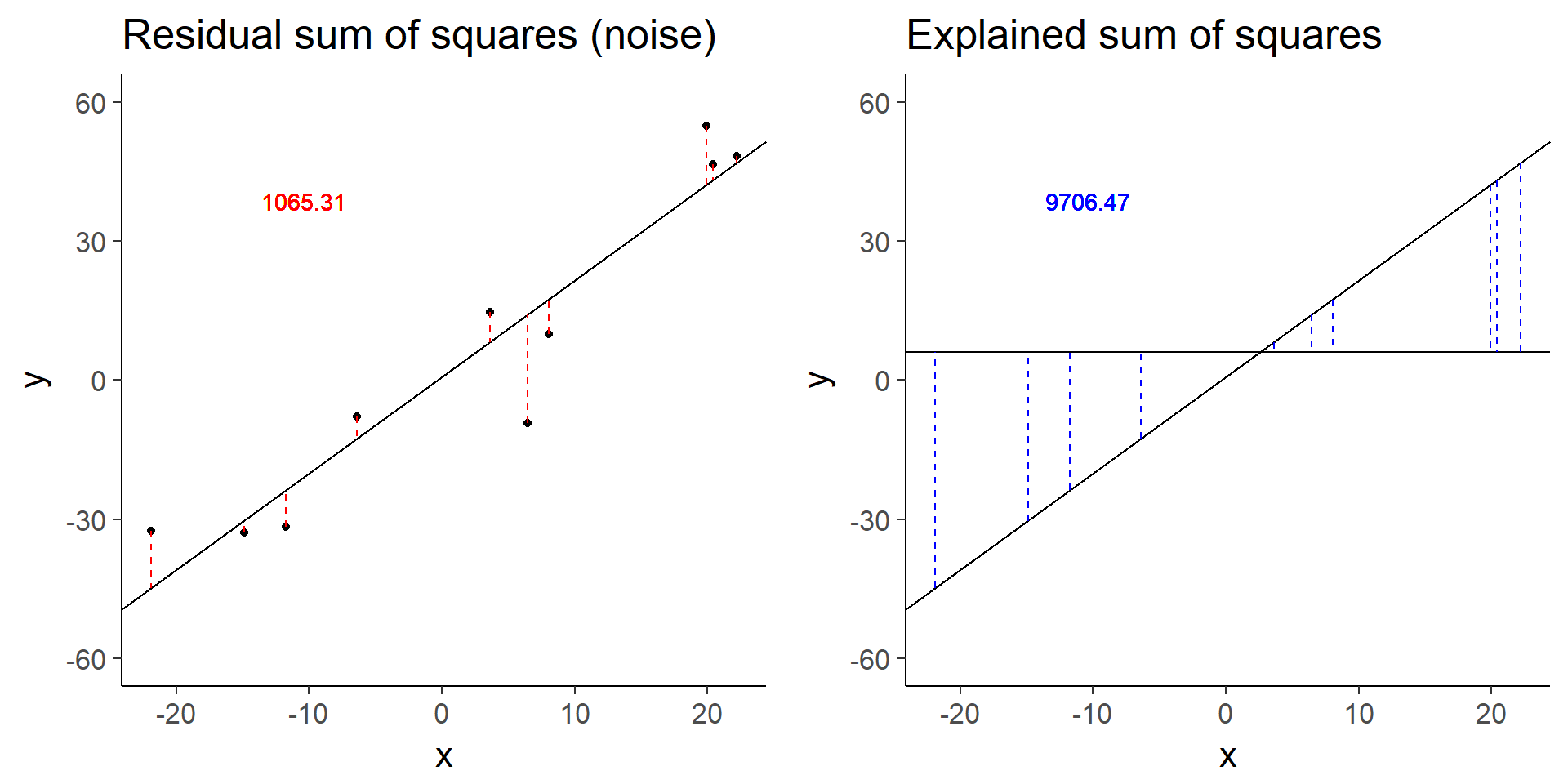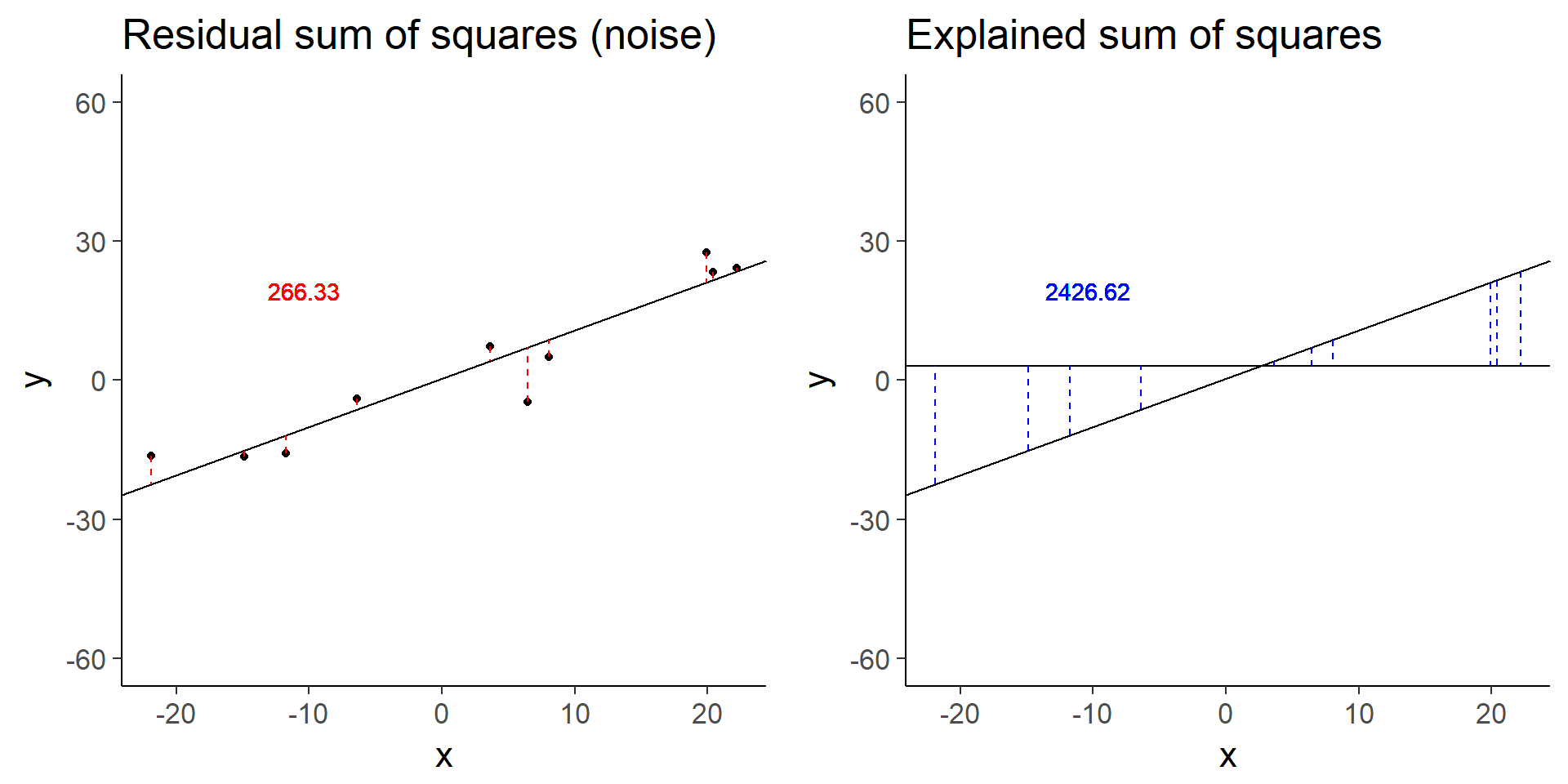Last updated: 2018-08-20

• R Markdown file: up-to-date

Great! Since the R Markdown file has been committed to the Git repository, you know the exact version of the code that produced these results.

• Environment: empty

Great job! The global environment was empty. Objects defined in the global environment can affect the analysis in your R Markdown file in unknown ways. For reproduciblity it’s best to always run the code in an empty environment.

• Seed: set.seed(12345)

The command set.seed(12345) was run prior to running the code in the R Markdown file. Setting a seed ensures that any results that rely on randomness, e.g. subsampling or permutations, are reproducible.

• Session information: recorded

Great job! Recording the operating system, R version, and package versions is critical for reproducibility.

• Repository version: fcc513c

Great! You are using Git for version control. Tracking code development and connecting the code version to the results is critical for reproducibility. The version displayed above was the version of the Git repository at the time these results were generated.

Note that you need to be careful to ensure that all relevant files for the analysis have been committed to Git prior to generating the results (you can use wflow_publish or wflow_git_commit). workflowr only checks the R Markdown file, but you know if there are other scripts or data files that it depends on. Below is the status of the Git repository when the results were generated:

Ignored files:
Ignored:    .Rhistory
Ignored:    .Rproj.user/

Untracked files:
Untracked:  code/counts_per_sample.txt
Untracked:  code/table-s1.txt


Note that any generated files, e.g. HTML, png, CSS, etc., are not included in this status report because it is ok for generated content to have uncommitted changes.
Expand here to see past versions:
File Version Author Date Message
html 4976490 John Blischak 2018-08-20 Build site.
Rmd 1920833 John Blischak 2018-08-20 Refactor first edition of chapter 1 into distinct lessons.

Introduction

The goal is to provide some visualizations to help you understand linear models.

Setup

library("broom")
library("cowplot")
library("dplyr")
library("ggplot2")
theme_set(theme_classic(base_size = 16))
library("knitr")
ss <- anova_tidy$sumsq ss_exp <- ss ss_res <- ss stopifnot(ss_exp - sum((y_hat - y_bar)^2) < 0.01) stopifnot(ss_res - sum(residuals(mod)^2) < 0.01) return(data.frame(x, y, y_bar, intercept, slope, y_hat, fstat, ss_exp, ss_res, row.names = 1:n)) } # Visualize the residual sum of squares plot_ss_res <- function(d) { ggplot(d, aes(x = x, y = y)) + geom_point() + geom_abline(aes(intercept = intercept, slope = slope)) + geom_linerange(aes(ymin = y, ymax = y_hat), color = "red", linetype = "dashed") + geom_text(aes(x = quantile(x, 0.25), y = quantile(y, 0.75), label = round(ss_res, 2)), color = "red") + labs(title = "Residual sum of squares (noise)") + ylim(-60, 60) } # Visualize the explained sum of squares plot_ss_exp <- function(d) { ggplot(d, aes(x = x, y = y)) + geom_abline(aes(intercept = intercept, slope = slope)) + geom_hline(aes(yintercept = y_bar)) + geom_linerange(aes(ymin = y_hat, ymax = y_bar), color = "blue", linetype = "dashed") + geom_text(aes(x = quantile(x, 0.25), y = quantile(y, 0.75), label = round(ss_exp, 2)), color = "blue") + labs(title = "Explained sum of squares") + ylim(-60, 60) } Simulation: Baseline # baseline baseline <- sim_lm(n = 10, effect = 2, error = 5) baseline_ss_res <- plot_ss_res(baseline) baseline_ss_exp <- plot_ss_exp(baseline) plot_grid(baseline_ss_res, baseline_ss_exp)Expand here to see past versions of baseline-1.png: Version Author Date 4976490 John Blischak 2018-08-20 baseline$fstat
 280.3557

The baseline simulation has an F-statistic of 280.3557372.

Simulation: Increased error

# Increased error
more_error <- sim_lm(n = 10, effect = 2, error = 10)
more_error_ss_res <- plot_ss_res(more_error)
more_error_ss_exp <- plot_ss_exp(more_error)
plot_grid(more_error_ss_res, more_error_ss_exp)Expand here to see past versions of increased-error-1.png:
Version Author Date
4976490 John Blischak 2018-08-20

more_error$fstat  72.89125 Doubling the error decreases the test statistic by a factor of 4. Simulation: Decreased signal # Decreased signal less_signal <- sim_lm(n = 10, effect = 1, error = 5) less_signal_ss_res <- plot_ss_res(less_signal) less_signal_ss_exp <- plot_ss_exp(less_signal) plot_grid(less_signal_ss_res, less_signal_ss_exp)Expand here to see past versions of decreased-signal-1.png: Version Author Date 4976490 John Blischak 2018-08-20 less_signal$fstat
 72.89125

Similarly, halving the signal decreases the test statistic by a factor of 4.

Session information

sessionInfo()
R version 3.5.0 (2018-04-23)
Platform: x86_64-w64-mingw32/x64 (64-bit)
Running under: Windows 10 x64 (build 17134)

Matrix products: default

locale:
 LC_COLLATE=English_United States.1252
 LC_CTYPE=English_United States.1252
 LC_MONETARY=English_United States.1252
 LC_NUMERIC=C
 LC_TIME=English_United States.1252

attached base packages:
 stats     graphics  grDevices utils     datasets  methods   base

other attached packages:
 bindrcpp_0.2.2 knitr_1.20     dplyr_0.7.5    cowplot_0.9.2
 ggplot2_2.2.1  broom_0.4.4

loaded via a namespace (and not attached):
 Rcpp_0.12.17      compiler_3.5.0    pillar_1.2.3
 git2r_0.21.0      plyr_1.8.4        workflowr_1.1.1
 bindr_0.1.1       R.methodsS3_1.7.1 R.utils_2.6.0
 tools_3.5.0       digest_0.6.15     gtable_0.2.0
 evaluate_0.10.1   tibble_1.4.2      nlme_3.1-137
 lattice_0.20-35   pkgconfig_2.0.1   rlang_0.2.1
 psych_1.8.4       yaml_2.1.19       parallel_3.5.0
 stringr_1.3.1     rprojroot_1.3-2   grid_3.5.0
 tidyselect_0.2.4  glue_1.2.0        R6_2.2.2
 foreign_0.8-70    rmarkdown_1.10    purrr_0.2.5
 tidyr_0.8.1       reshape2_1.4.3    magrittr_1.5
 whisker_0.3-2     scales_0.5.0      backports_1.1.2
 htmltools_0.3.6   assertthat_0.2.0  mnormt_1.5-5
 colorspace_1.3-2  labeling_0.3      stringi_1.2.3
 lazyeval_0.2.1    munsell_0.5.0     R.oo_1.22.0      

This reproducible R Markdown analysis was created with workflowr 1.1.1